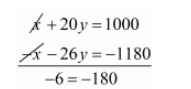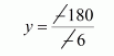# A part of monthly hostel charges in a college are fixed and the remaining depend on the number of days one has taken food in the mess.`
Question:

A part of monthly hostel charges in a college are fixed and the remaining depend on the number of days one has taken food in the mess. When a student A takes foods for 20 days, he has to pay Rs 1000 as hostel charges whereas a students B, who takes food for 26 days, pays Rs 1180 as hostel charges. Find the fixed charge and the cost of food per day.

Solution:

Let the fixed charges of hostel be $R s . x$ and the cost of food charges be $R s . y$ per day

According to the given condition we have,

$x+20 y=1000 \cdots(i)$

$x+26 y=1180 \cdots(i i)$

Subtracting equation $(i i)$ from equation $(i)$ we get$y=30$

Putting $y=30$ in equation $(i)$ we get

$x+20 y=1000$

$x+20 \times 30=1000$

$x+600=1000$

$x=1000-600$

$x=400$

Hence, the fixed charges of hostel is Rs 400

The cost of food per day is Rs 30